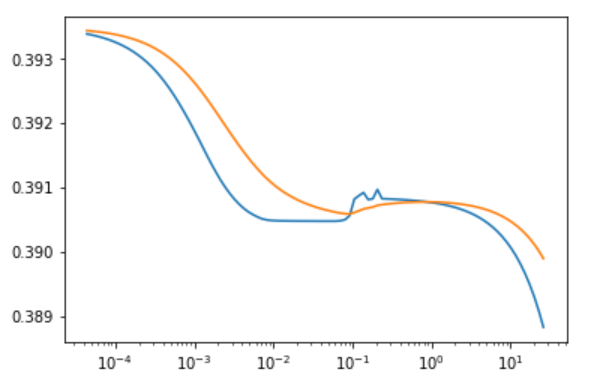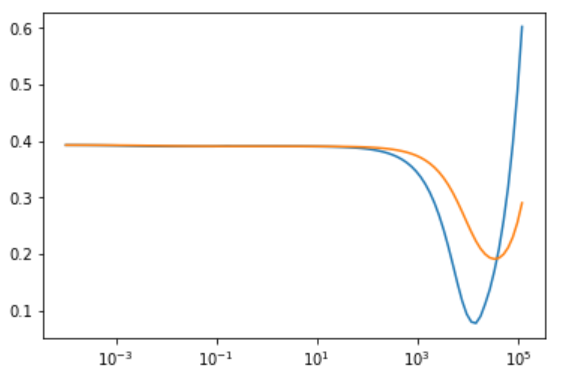Karpathy wrote Convnetjs and in one demo showed how to learn an image via regression.

In here the two linear layers are used instead and single ReLU in between so the model looks like this:

model = torch.nn.Sequential(
torch.nn.Linear(90000, 100),
torch.nn.ReLU(True),
torch.nn.Linear(100, 90000)
)


The image was 150x200 pixels big.The whole work is in this gist.

As you may see experimenting with the learning rate showed me interesting things:The best learning rate (x-axis) would be probable around 1e3, although you may not even try this at first since usually people experiment with the learning rates smaller than 1.

However, trying the range up to 1e5 showed me the bigger learning rates will just work.

In here the torch.nn.functional.l1_loss loss function was used, but other would work as well.

I could use any of the:

inp = t.reshape(-1)[None].cuda()
z = torch.zeros(1,90000).cuda()
r = torch.rand(1,90000).cuda()


where t is tensor of the image to be learned. This can be interpreted in order to learn I could use any input, including the image to be learned itself.